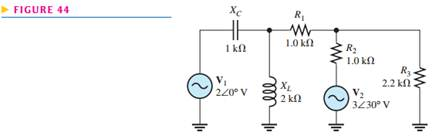### Create an Account

Home / Questions / Using the superposition method calculate the current through R3 in Figure 44

# Using the superposition method calculate the current through R3 in Figure 44

Using the superposition method, calculate the current through R3 in Figure 44.Jul 31 2020 View more View LessSubscribe To Get Solution